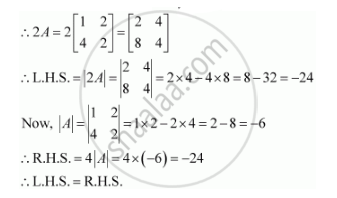Share

# If a = [(1,2),(4,2)] Then Show that |2a| = 4|A| - CBSE (Commerce) Class 12 - Mathematics

ConceptDeterminants of Matrix of Order One and Two

#### Question

if A = [(1,2),(4,2)] then show that |2A| = 4|A|

#### Solution

The given matrix is A = [(1,2),(4,2)]Is there an error in this question or solution?

#### Video TutorialsVIEW ALL 

Solution If a = [(1,2),(4,2)] Then Show that |2a| = 4|A| Concept: Determinants of Matrix of Order One and Two.
S